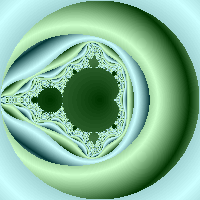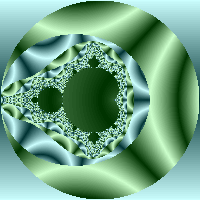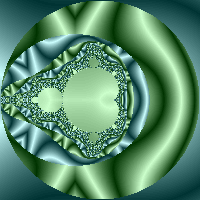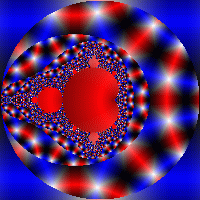## Coloring Techniques

The purpose of this document is to explain a number of fractal coloring options. In reality the number of available coloring techniques is infinite but here we'll just go through a representative sample to provide a basis for futher experimentation.

We'll be using the same formula file throughout this discussion - a simple mandelbrot fractal. The portions of the file that will not change are as follows:

```   fractal
{
mapping
{
(-2, -2, 2, 2) => (200, 200)
}

// 'colors' section (if used) will go here

formula
{
z = [0, 0];

while(\$count < 100 && ssq(z) < 4)
{
z = z * z + current;
}

// color assignment will go here
}
}
```

There are two ways to assign color:

• indexed color (using a color table)
• direct color (specify an rgb value)

Here is a minimal example that assigns the color red using an indexed color model. This example uses the formula above, its colors section is:

```colors
{
define
{
[255 0 0] // this is entry 0
[0 255 0] // this is entry 1
[0 0 255] // this is entry 2
}
}
```
And it's color assigment is:
```set_color(0);
```

Note that the value passed to set_color (in this case zero) is what is used to select a color from the color table. If we had wanted to assign a color of blue instead, we would have used:

```set_color(2);
```

Now here is an equally straightforward example that also assigns the color red but this time using a direct color approach

```// No need for a 'colors' section when using direct color

set_color(255, 0, 0);
```

In practice, of course, we wouldn't use the same value each time - this would result in a pretty uninteresting image (a solid color). When doing real color assignment we would compute the index (when doing color-table based color) or rgb value (when doing direct color). It is the flexibility allowed in the computation that allows us to color our fractal images in so many different ways.

Now we'll examine some "real world" examples, we'll cover indexed (color table based) color assignment first.

#### coloring techniques - indexed color

One very important point about using indexed color is this:

Care must be taken to ensure that the calculation you use to generate the appropriate color index falls within the bounds of the color table you have created

While this is important, the program is forgiving - if you supply an index that is outside the bounds of your color table, that pixel will simply be colored black and you will see a brief message once your image has been fully drawn detailing how many invalid indices were encountered while processing your image.

Now we'll cover some common techniques:

• Coloring based upon the angle of 'z' upon bailout

• Colors section
```   colors
{
define
{
{
[0 0 0]
[255 255 255]
[0 0 0]
}
}
}
```

• Color assignment statements
```      set_color(deg(z));
```

• Resulting image• Explanation

• The 'gradient' command creates a series of 361 colors (for more detail see this) that specify a smooth transition from black to white then back to black again.

• The 'set_color' command selects an index from the color table corresponding the the angle (in degrees) that 'z' made with the origin when the while loop terminated (for more detail see this). Note that our color table defines entries from 0 to 360 so there is a corresponding entry for each angle in degrees (we won't generate any 'out of bounds' entries)

• Coloring based upon the angle of 'z' upon bailout using two gradients

• Colors section
```   colors
{
define
{
{
[204 255 255]
[0 51 51]
[204 255 255]
}
}
define(360)
{
{
[204 255 204]
[0 51 0]
[204 255 204]
}
}
}
```

• Color assignment statements
```      if(fmod(\$count, 2) == 0)
{
set_color(deg(z));
}
else
{
set_color(360 + deg(z));
}
```

• Resulting image• Explanation

• Here we set up two gradients, each covering 360 indices (one per degree). The cyan shades cover (0 - 359) and the green shades color (360 - 719)

• We want to use the cyan set half the time and the green set half the time so we use \$count (the number of iterations of the while loop that were executed before the loop terminated) for this purpose. The statement:
```   if(fmod(\$count, 2) == 0)
```
Says "if count was even", the reason being that if \$count was an even number then the remainder when dividing it by two will be zero. Note that when \$count is odd, we add 360 to the z's angle to select the portion of our color table containing the green entries.

It is also worth noting that we could have done an equivalent color assignment in a number of other ways, such as:

• ```   \$value = deg(z);

if(fmod(\$count, 2) == 1)
{
\$value = \$value + 360;
}

set_color(\$value);
```
• ```   set_color(fmod(\$count, 2) * 360 + deg(z));
```
The resulting value passed to set_color is all that matters - the manner in which we generate the value is up to us.

• Coloring based upon the angle of 'z' upon bailout using three gradients

• Colors section
```   colors
{
define
{
{
[0 51 0]
[204 255 204]
[0 51 0]
}
}

define(360)
{
{
[0 51 51]
[204 255 255]
[0 51 51]
}
}

define(720)
{
{
[204 153 0]
[255 255 204]
[204 153 0]
}
}
}
```

• Color assignment statements
```      \$value = 0;

if(fmod(\$count, 3) == 1)
{
\$value = 360;
}
else
{
if(fmod(\$count, 3) == 2)
{
\$value = 720;
}
}
set_color(\$value);

```

• Resulting image• Explanation

• This example is just an extension of the previous, now we have three sets of colors instead of two. The ranges are:

0 - 359green
360 - 719cyan
720 - 1079yellow

• When we use fmod with 3, we'll end up with a result of 0, 1 or 2. We use these resulting values to offset the z's angle by 0, 360 or 720 to select the appropriate color set

• Coloring based upon the magnitude of 'z'

• Colors section
```   colors
{
define
{
{
[0 51 0]
[204 255 204]
[0 51 0]
}
}

define(100)
{
{
[0 51 51]
[204 255 255]
[0 51 51]
}
}
}
```

• Color assignment statements
```      \$value = fmod(mag(z), 5) * 20;

if(fmod(\$count, 2) == 1)
{
\$value = \$value + 100;
}

set_color(\$value);

```

• Resulting image• Explanation

• The color table used here uses two gradients - note that the size of each gradient here is 101, not 361 as in the previous examples. The reason we used 361 before is because before we were working with angles (which range from 0 to 360), in this magnitude based example we just chose 100 as that size results in a nice looking range of colors.

• The magnitude of a complex number can really be anything - it is the distance of the value from the origin (for more details see this) so we need to restrict its range to ensure the value we produce isn't outside the bounds of our color table. My doing:
```         fmod(mag(z), 5)
```
We get a value between 0 and 5 which we then multiply by 20 to produce a value between 0 and 100. Once we have that value, we use \$count to alternately choose betwen the first and second set of colors.

#### more indexed color techniques

Now that we've seen a number of the standard coloring methods, we'll quickly cover some additional variants just to give you some ideas for your own experimentation. For these examples, the color section will be fixed:

```   colors
{
define
{
{
[0 51 0]
[204 255 204]
[0 51 0]
}
}

define(100)
{
{
[0 51 51]
[204 255 255]
[0 51 51]
}
}
}
```

We'll just show the color assignment section and resulting images so that we can cover a number of ideas rapidly.

• color assignment
```\$value1 = 0;
\$value2 = 0;

if(fmod(\$count, 2) == 0)
{
\$value1 = abs(real(z));
}
else
{
\$value1 = abs(imag(z));
\$value2 = 100;
}

set_color(fmod(\$value1, 4) * 25 + \$value2);
```
• image• color assignment
```\$value = abs(sin(real(z) * imag(z))) * 100;

if(fmod(\$count, 2) == 1)
{
\$value = \$value + 100;
}

set_color(\$value);
```
• image• color assignment
```z_power = pow(z, [imag(z), real(z)]);
\$value = deg(z_power) * (100 / 360);

if(fmod(\$count, 2) == 1)
{
\$value = \$value + 100;
}

set_color(\$value);
```
• imageCertainly some of the above techniques seem complicated, but their purpose is just to illustrate how many coloring possibilities are available. The best way to develop new techniques is just to experiment, there are an unlimited number of interesting and beautiful coloring approaches waiting to be explored.

#### direct color based techniques

With direct color assignment, you do not need a colors section (though it is not an error to have one). Direct color assignment just involves generating values for red, green and blue - each between 0 and 255.

If any of your generated values are less than 0 or greater than 255 the pixel will be colored black and (much like indexed color assignment) you will receive a notification when you image has fully drawn indicating the number of invalid color assignments.

Also note that direct and indexed color are not mutually exclusive alternatives - there is no problem with mixing them together within the same generated image.

Here is an example using direct color assignment:

• (not needed)

• Color assignment statements
```      \$value1 = abs(sin(real(z)));
\$value2 = abs(cos(imag(z)));

\$red   = 255 - 255 * \$value1;
\$blue  = 255 - 255 * \$value2;
\$green = min(\$red, \$blue);

set_color(\$red, \$green, \$blue);
Resulting imageExplanation

Consider the first two calculations:
\$value1 = abs(sin(real(z)));
\$value2 = abs(cos(imag(z)));

The sin or cos of any number will be a value between -1 and 1.  Taking the
absolute value gives us a value between 0 and 1 for both \$value1 and \$value2.

Our goal here is to assign to \$red, \$green and \$blue a value between 0 and
255.  We might have assigned \$red and \$blue more simply as:
\$red   = 255 * \$value1;
\$blue  = 255 * \$value2;